Courses

# Worksheet Questions - Quadratic Equations Class 10 Notes | EduRev

## Class 10 : Worksheet Questions - Quadratic Equations Class 10 Notes | EduRev

The document Worksheet Questions - Quadratic Equations Class 10 Notes | EduRev is a part of the Class 10 Course Mathematics (Maths) Class 10.
All you need of Class 10 at this link: Class 10

1. A natural number, when increased by 12, equals 160 times its reciprocal. Find the number.
Hint: Let the natural number be x
∴ x + 12 = 160/x
⇒ x(x + 12) = 160
⇒ x2 + 12x – 160 = 0

2. By increasing the list price of a book by ₹ 10, a person can buy 10 books less for ₹ 1200. Find the original list price of the book.

3. The hypotenuse of a right-angled triangle is 1 cm more than twice the shortest side. If the third side is 2 cm less than the hypotenuse, find the sides of the triangle.

4. A passenger train takes 2 hours less for a journey of 300 km, if its speed is increased by 5 km/hr from its usual speed. Find its usual speed.

5. The numerator of a fraction is one less than its denominator. If three is added to each of the numerator and denominator, the fraction is increased by 3/28. Find the fraction.

6. The difference of squares of two natural numbers is 45. The square of the smaller number is four times the larger number. Find the numbers

7. Solve: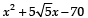8. A train travels a distance of 300 km at a uniform speed. If the speed of the train is increased by 5 km an hour, the journey would have taken two hours less. Find the original speed of the train.

9. The speed of a boat in still water is 11 km/ hr. It can go 12 km upstream and returns downstream to the original point in 2 hours 45 minutes. Find the speed of the stream.

10. Determine the value of k for which the quadratic equation 4x2 - 3kx + 1 = 0 has equal roots.

11. Using quadratic formula, solve the following equation for ‘x’:
ab x2 + (b2 - ac) x - bc = 0

12. The sum of the numerator and the denominator of a fraction is 12. If the denominator is increased by 3, the fraction becomes 1/2. Find the fraction.

13. Rewrite the following as a quadratic equation in x and then solve for x: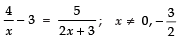14. A two digit number is such that the product of its digits is 18. When 63 is subtracted from the number, the digits interchange their places. Find the number.

15. A train covers a distance of 90 km at a uniform speed. Had the speed been 15 km/ hr more, it would have taken 30 minutes less for the journey. Find the original speed of the train.

16. Solve for x: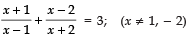17. Using quadratic formula, solve the following for x: 9x2 − 3 (a2 + b2) x + a2 b2 = 0

18. Find the equation whose roots are reciprocal of the roots of 3x2 − 5x + 7 = 0

19. A number consists of two digits whose product is 18. When 27 is subtracted from the number, the digits change their places.

Find the number.

20. A number consisting of two digits is seven times the sum of its digits. When 27 is subtracted from the number, the digits are reversed. Find the number.

21. The sum of the squares of two consecutive odd numbers is 394. Find the nimbers.
Hint:

Let the two consecutive odd numbers be x and x + 2.

∴ x2 + (x + 2)2 = 394

⇒ x2 + x2 + 4x + 4 = 394

⇒ 2x2 + 4x – 390 = 0

⇒ x2 + 2x – 195 = 0
⇒ x2 + 15x – 13x – 195 = 0
or
x(x + 15) – 13(x + 15) = 0
⇒ x= 13 or x = – 15
∴  For  x = 13, x + 2 = 13 + 2 = 15
Thus, the required numbers are 13 and 15.

22. An aeroplane left 30 minutes later than its scheduled time and in order to reach its destination 1500 km away in time, it has to increase its speed by 250 km/hr from its usual speed. Determine its usual speed.

23. Using quadratic formula, solve for x:

24. Find the number which exceeds its positive square root by 20.

25. The sum of two numbers is 15 and the sum of their reciprocals is 1/3. Find the numbers.

26. A two digit number is such that the product of its digits is 14. If 45 is added to the number, the digits interchange their places.

Find the number.

27. A two digit number is such that the product of its digits is 20. If 9 is added to the number, the digits interchange their places. Find the number.

28. A two digit number is such that the product of its digits is 15. If 8 is added to the number, the digits interchange their places. Find the number.

29. Solve for x :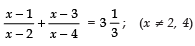30. Solve for x: ab x2 + (b2 − ac) x − bc = 0

31. The sum of two numbers is 18. The sum of their reciprocals is 1/4.  Find the numbers.

33. The sum of two numbers ‘a’ and ‘b’ is 15, and sum of their reciprocals 1/a and 1/b is 3/10. Find the numbers ‘a’ and ‘b’.

34. Solve for x: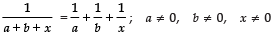35. Find the roots of the following quadratic equation: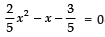Hint: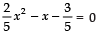⇒ 2x2 − 5x − 3 = 0
⇒ (2x + 1) (x − 3) = 0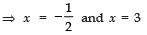36. Find the roots of the equation: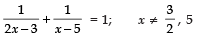37. A natural number when subtracted from 28, becomes equal to 160 times its reciprocal.

Find the number.

38. Find two consecutive odd positive integers, sum of whose squares is 290.

39. Find the values of k for which the quadratic equation
(k + 4) x2 + (k + 1) x + 1 = 0 has equal roots.

Also find these roots.
Hint: From (k + 4)x2 + (k + 1)x + 1 = 0, we get
a = (k + 4),b = (k + 1)  and c = 1
∴ b2 – 4ac = (k + 1)2 – 4 (k + 4) (1)
= k2 – 2k – 15
For equal roots, b2 – 4ac = 0
∴ k2 – 2k – 15 = 0
⇒ k = 5 or k = – 3
For k = 5, we have (k + 4)x2 + (k + 1) x + 1 = 0

≡ 9x2 + 6x + 1 = 0
Solving for x, we get x = -1/3, -1/3
For k = – 3, we have
x2 – 2x + 1 = 0
∴ solving it, we get x = 1, x = 1

40. Solve for x :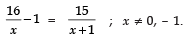Hint: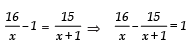or
16(x + 1) – 15(x) = x(x + 1) ⇒ x2 = 1 6
x = ± 4

41. Solve for x :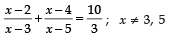Hint: L.H.S.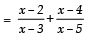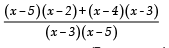(By cross multiplication)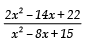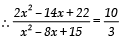or
3[2x2 – 14x + 22]
= 10 [x2 – 8x + 15]
On simplification, we get
2x2 – 19x + 42 = 0 ⇒ x = 7/2

42. Find the value of k, for which one root of quadratic equation kx2 – 14x + 8 = 0 is six times the other.

43. If x =  2/3 and x = –3 are roots of the quadratic equation ax2 + 7x + b = 0, find the value of a and b.

44. If –5 is a root of the quadratic equation 2x2 + px – 15 = 0 and the quadratic equation p (x2 + x) + k = 0 has equal roots, find the value of k.

45. Find x in terms of a, b and c :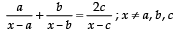Hint :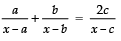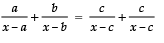ANSWERS
1.

2. ₹ 30

3. 8 cm, 15 cm, 17 cm

4. 25 km/hr

5. 3/4

6. 9 and 6

7.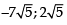8. 25 km/hr

9. 5 km/hr

10.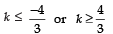11.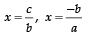12. 5/7

13. –2, 1

14. 92

15. 45 km/hr

16. x = –5, 2

17.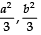18. 7x2 – 5x + 3 = 0

19. 63

20. 63

21. 14

22. 750 km/h

23. x = a/3 , b/3

24. x = 16

25. 5, 10

26. 27

27. 45

28. 35

29.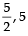30. x = –b

31. 6, 12

32. 12, 14

33. a = 5 or 10, b = 10  or 5

34. x = –a

35.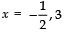36.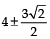37.

38. 11 and 13

42. k = 3

43. a = 3, b = –6

44. k = 7/4

45.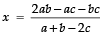Offer running on EduRev: Apply code STAYHOME200 to get INR 200 off on our premium plan EduRev Infinity!

## Mathematics (Maths) Class 10

62 videos|363 docs|103 tests

,

,

,

,

,

,

,

,

,

,

,

,

,

,

,

,

,

,

,

,

,

;